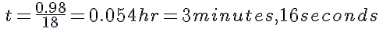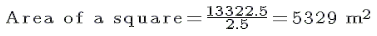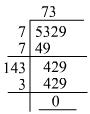# RD Sharma Solutions for Class 8 Math Chapter 3 - Squares and Square Roots (Part-4) Notes | Study RD Sharma Solutions for Class 8 Mathematics - Class 8

## Class 8: RD Sharma Solutions for Class 8 Math Chapter 3 - Squares and Square Roots (Part-4) Notes | Study RD Sharma Solutions for Class 8 Mathematics - Class 8

The document RD Sharma Solutions for Class 8 Math Chapter 3 - Squares and Square Roots (Part-4) Notes | Study RD Sharma Solutions for Class 8 Mathematics - Class 8 is a part of the Class 8 Course RD Sharma Solutions for Class 8 Mathematics.
All you need of Class 8 at this link: Class 8

#### Hence, the length of the square field is 245 m.The square has four sides, so the number of boundaries of the field is 4.The distance s covered by the man = 245 m ×× 4 = 980 m = 0.98 kmIf the velocity v is 18 km/hr, the required time t can be calculated using the following formula:t= s/v#### Answer 10: First, we have to find the area of the square lawn, which the total cost divided by the cost of levelling and turfing per square metre:The length of one side of the square is equal to the square root of the area. We will use the long division method to find it as shown below:Length of one side of the square = 73 m
The circumference of the square is 73 ×× 4 = 292 m
Total cost of fencing the lawn at Rs. 5 per metre = 292 ×× 5 = Rs. 1460

#### 23000 is 4 (704 − 700) less than 482. Hence, 4 must be added to 2300 to get a perfect square.

The document RD Sharma Solutions for Class 8 Math Chapter 3 - Squares and Square Roots (Part-4) Notes | Study RD Sharma Solutions for Class 8 Mathematics - Class 8 is a part of the Class 8 Course RD Sharma Solutions for Class 8 Mathematics.
All you need of Class 8 at this link: Class 8Use Code STAYHOME200 and get INR 200 additional OFF

## RD Sharma Solutions for Class 8 Mathematics

88 docs

Track your progress, build streaks, highlight & save important lessons and more!

,

,

,

,

,

,

,

,

,

,

,

,

,

,

,

,

,

,

,

,

,

;